Types of Angles

Types of Angles - Acute, Right, Obtuse, Straight and Reflex Anlges

When two lines intersect, at the point of their intersection an angle is formed. Learning about angles is important, as they form the base of geometry. The two rays that form the angle are known as the sides of the angle. Also, it is not necessary that an angle is formed by intersection of two straight lines; it can be formed by intersection of two curved lines too.

There are various types of angles based on their measure of the angle. The types are,

• 1. Acute angle

• 2. Right angle

• 3. Obtuse angle

• 4. Straight angle

• 5. Reflex angle

• Types of angles

• 1. Acute angle

• An angle which measures less than 90° is called an acute angle. The measure between 0° to 90°. In the picture below, the angle formed by the intersection of PQ and QR at Q forms an angle PQR which measures 45°. Thus, PQR is called an acute angle.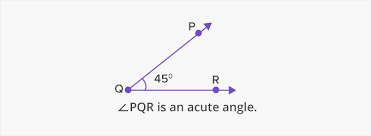• 2. Right angle

• An angle which measures exactly 90° is called a right angle. It is generally formed when two lines are perpendicular to each other. In the figure below, line AB intersects line BC at B and form an angle ABC which measures 90°.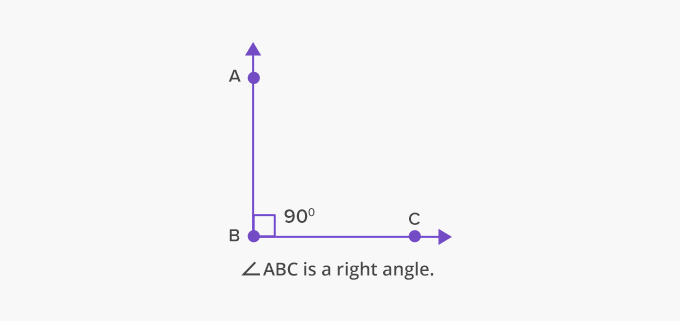• 3. Obtuse angle

• An angle that measures greater than 90° is known as the obtuse angle. The angle measure ranges from 90° to 180°. An obtuse angle can also be found out if we have the measure of the acute angle.

Obtuse angle measure = (180- acute angle measure)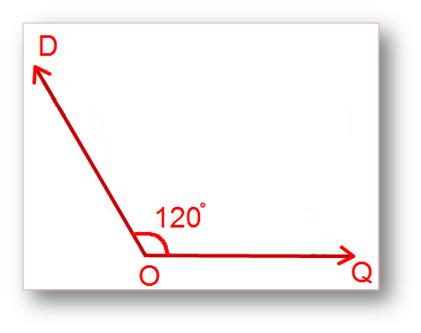In the picture above, line segment DO intersect line segment OQ at point O and forms an angle DOQ measuring 120°. Thus, it is an obtuse angle.

Also, if we extend line OQ to OP then we can find a measure of the acute angle.

DOP = 180° - DOQ = 180° - 120° = 60°

• 1. Straight angle

• The angle which measures exactly 180° is called a straight angle. This is similar to a straight line, thus the name straight angle.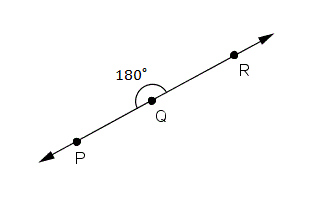A straight angle is nothing but a mixture of an obtuse angle and acute angle on a line.
• 2. Reflex angle

• The angle which measures greater than 180° and less than 360° is known as the reflex angle. The reflex angle can be calculated if the measure of the acute angle is given, as it is complementary of the acute angle on the other side of the line.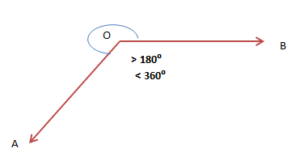Using the reflex angle, we can find the measure of the acute angle.
A measure of acute angle = 360° –a measure of reflex angle

Angle types

• 1. Complementary angle

• If two angles add up to measure 90° then they are known as complementary angles. The angles don't have to be adjacent to each other to be known as complementary. As long as they add up to 90° they will be known as complementary angles.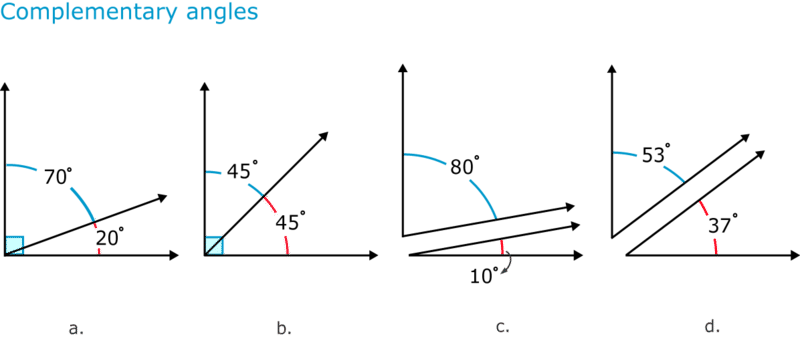In the figure, a and b the angles are present adjacent to each other and add up to 90° and thus are known as complementary angles. In figure c and d, the angles are not adjacent to each other, but they add up to 90° and thus they are known as complementary angles.

• 2. Supplementary angles

• When two angles add up to 180 ° then they are known as supplementary angles. There are various types of supplementary angles.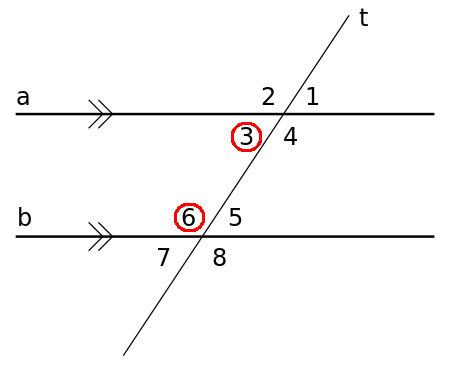• a. Vertical Angles

• Angles which have a common vertex and the sides of the angle are formed by the same lines are known as vertical angles. Vertical angles are equal to each other.

In the above figure, 1 and 3, 2 and 4, 6 and 8 and 5 and 7 are vertical angles. Also, 3, 4,5, 6 are known as interior angles and 1,2,7,8 are known as exterior angles.

• b. Alternate interior angles

• These are a pair of interior angles present on the opposite side of the transversal. The easiest way to spot alternate interior angles is to identify a "Z” on the interior side.
In the above figure, 3 and 5, 4 and 6 are interior angles. The interior angles equal to one another.

• c. Alternate exterior angles

• This is similar to alternate interior angles; just that it is present on the exterior side. In the above figure, 1 and 7, 2 and 8 are the pair of alternate exterior angles. Similar to alternate interior angles, even alternate exterior angles are equal to one another.

• d. Corresponding Angles

• Angles which are present in a similar position are known as corresponding angles. In the above figure, 1 and 5 are corresponding angles and they are equal to one another.

Summary
 Angle type Angle measure Acute angle Greater than 0 °, Less than 90° Right angle 90° Obtuse angle Greater than 90°, less than 180° Straight angle 180° Reflex angle Greater than 180°, less than 360°

The major basis of geometry is angles. Angles finds its application in nearly all types of questions, be it trigonometry to closed shapes. Understanding angles and angle types will help in solving a lot of tricky questions. Thus, make sure that you understand it well.